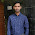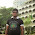## Electrical Circuit Theory Solved Objective Questions asked in GATE, IES:

 The period of the signal x(t) = 8 sin (0.8πt + π /4) is [GATE 2010]
A. 0.4πs
B. 0.8πs
C. 1.25s
D. 2.5s

 The switch in the circuit has been closed for a long time. It is opened at t=0. At t=0+,the current through the 1μF capacitor is [GATE 2010]
A. 0A
B. 1A
C. 1.25A
D. 5A

 The second harmonic component of the periodic waveform given in the figure has an amplitude of [GATE 2010]
A. 0
B. 1
C. 2/π
D. √5

 As shown in the figure, a 1 resistance is connected across a source that has a load line v+i=100. The current through the resistance is [GATE 2010]
A. 25A
B. 50A
C. 100A
D. 200A

 If the electrical circuit of figure (b) is an eqiuvalent of the coupled tank system of figure (a), then [GATE 2010]

A. A,B are resistances and C,D capacitances
B. A,C are resistances and B,D capacitances
C. A,B  are capacitances and C,D resistances
D. A,C are capacitances are and B,D resistances

 If the 12Ω resistor draws a current of 1A as shown in the figure, the value of resistance R is [GATE 2010]
A. 4Ω
B. 6Ω
C. 8Ω
D. 18Ω

 The two-port network P shown in the figure has ports 1 and 2, denoted by terminals (a,b) and (c,d), respectively. It has an impedence matrix Z with parameters denoted by Zij. A 1Ω resistor is connected in series with the network at port 1 as shown in the figure. The impedance matrix of the modified two-port network (shown as a dashed box) is [GATE 2010]The Maxwell's bridge shown in the figure is at balance.The parameters of the inductive coil are [GATE 2010]

A. R=R2R3/R4, L=C4R2R3
B. L=R2R3/R4, R=C4R2R3
C. R=R4/R2R3, L=1(C4R2R3)
D. L=R4/R2R3, R=1/(C4R2R3)

Statement for Q9 & Q10:
The L-C circuit shown in the figure has an inductance L=1mH and a capacitance C=10μF
Question : The initial current through the inductor is zero, while the initial capacitor voltage is 100V. The switch is closed at t=0. The current i through the circuit is: [GATE 2010]
A. 5cos(5x103t)A
B. 5sin(104t)A
C. 10cos(5x103t)A
D. 10sin(104t)A

Question : The L-C circuit of statement is used to commutate a thyristor, which is initially carrying a current of 5A as shown in the figure below. The values and initial conditions of L and C are the same as in statement. The switch is closed at  t=0. If the forward drop is negligible, the time taken for the device to turn off is [GATE 2010]

A. 52μs
B. 156μs
C. 312μs
D. 26μs

 The voltage applied to a circuit is 100√2 cos(100πt) volts and the circuit draws a current of  10√2sin(100πt +π/4) amperes. Taking the voltage as the reference phasor, the phasor representation of the current in amperes is [GATE 2011]
A. 10√2∠-  π/4
B. 10∠-  π/4
C. 10∠+ π/4
D. 10√2∠+ π/4

3:59 PM

#### 5 comments:

1.Solutions pls sir, q & a available everywhere but no explanation

2.Answer is D for the Question No.7

3.For 11 th qustn and is B

4.For 7th ans is D

5.For 11 qustn and is B